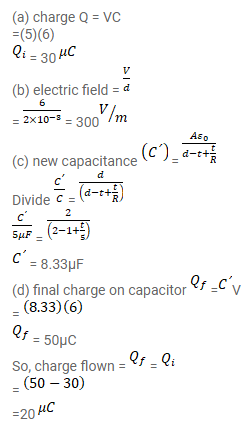# A parallel-plate capacitor of capacitance 5 μF is connected to a battery of emf 6 V.Question:

A parallel-plate capacitor of capacitance $5 \mu \mathrm{F}$ is connected to a battery of emf $6 \mathrm{~V}$. The separation between the plates is 2 $\mathrm{mm}$.

(a) Find the charge on the positive plate;

(b) Find the electric field between the plates,

(c) A dielectric slab of thickness 1 $\mathrm{mm}$ and dielectric constant 5 is inserted into the gap to occupy the lower half of it. Find the capacitance of the new combination,

(d) How much charge has flown through the battery after the slab is inserted?

Solution: